#Quality Digest

Featured Product
This Week in Quality Digest Live
Operations Features
Master Gage and Tool Co.
Why it matters for accurate measurements
Ian Wright
MIT and ETH Zurich engineers use computer vision to help adjust material deposition rates in real time
Etienne Nichols
It’s not the job that’s the problem. It’s the tools you have to do it with.
Lee Simmons
Lessons from a deep dive into 30 years of NFL and NBA management turnover
NIST
Having more pixels could advance everything from biomedical imaging to astronomical observations
Operations News
EcoBell paints plastic parts with minimal material consumption
Modern manufacturing execution software is integral for companies looking to achieve digital maturity
Study of intelligent noise reduction in pediatric study
Easy to use, automated measurement collection
A tool to help detect sinister email
Funding will scale Aigen’s robotic fleet, launching on farms in spring 2024
3D printing technology enables mass production of complex aluminum parts
High-end microscope camera for life science and industrial applications
Pretreatment rethought: EcoProWet PT
Operations

## \$EE, the Financial Aspect of OEE

### Reestablish communications between the front office and the shop floor

Published: Thursday, September 17, 2015 - 15:09Darin MarcuzLaron Colbert

This article describes a novel approach to calculating the financial aspect of overall equipment effectiveness (OEE), with the result referred to as \$EE (as in monetary units). By using \$EE, a management team readily can “SEE” their operation in financial terms. Employees are then better able to focus on underperforming operations to improve the bottom line.

The formula for calculating OEE is straightforward, and the product of factors (with units in per cent) is a very useful metric. In modern manufacturing, challenges arise when setting cycle time targets, measuring quality, or objectively classifying downtime. When these problems have been overcome, companies then face the issue of where to direct resources to improve the bottom line. The solution is to use \$EE.

### Background and practical limitations of OEE

In many businesses there is a communication breakdown between the front office and the shop floor which arises by coincidence. Because the three OEE production metrics (performance, yield, and availability) are not expressed in monetary units, daily efforts to improve processes using OEE alone do not always translate into significant bottom-line savings.

Of course it is possible to identify improvement projects which will significantly affect the bottom line, and then use control charts to measure significant inputs and outputs. Or, in the case of a customer complaint where the cost of quality is well understood, the problem is typically addressed by using structured problem-solving methods. Both of these examples illustrate a management-driven approach to problem solving where costs are calculated and resources are allocated.

Because the management team must focus on the financial picture and customer concerns, priorities can shift rapidly, sometimes overshadowing chronic issues which nevertheless must be addressed. At the same time, employees on the shop floor who are caught up in day-to-day production issues might be unaware of monthly or quarterly business trends. The way to bridge the gap in perspectives is through \$EE, the financial aspect of OEE.

The basic equation for OEE, from the ASQ Online Quality Glossary, can be stated as:

OEE = Performance efficiency × First-pass yield × Availability

Becuase the three factors which make up the equation are expressed as a percentage, the unit of the product is a percentage. Therefore OEE acts as a simple yet very powerful production gauge, wherein gains or losses in performance, yield, and/or availability result in a higher or lower percentage.

But what is the significance, from a financial perspective, when OEE changes? As mentioned earlier, this question is usually answered obliquely through a problem-solving approach whereby costs are understood up front and OEE is calculated afterwards. However on a day-to-day basis, when OEE is measured on individual or dissimilar processes, the question of financial significance remains unanswered.

The solution is to calculate the financial aspect of OEE, namely \$EE. By using budgetary amounts for machine overhead, scrap, and labor directly in equations which relate to production operations, OEE percentage can be translated into units of currency.

In the case of daily operations, especially when OEE is less than 100%, there is always room for improvement. Given enough time, resources, money (and justification), as the name implies, continuous improvement never ends. Additionally, when dealing with complex processes where OEE calculations have been applied, managers are continuously challenged to make the best use of resources strategically to improve the bottom line.

### \$EE, the financial aspect of OEE

When measuring OEE, it is important to scrutinize all data-collection systems which underlie the factors used in the calculation (performance, yield, and availability). The same applies for the calculation of \$EE, which uses the three OEE factors in addition to budgetary inputs. As long as the management team defines how each aspect of OEE and \$EE data is collected and calculated, then the journey of improvement can begin without roadblocks.

The financial aspect of OEE is defined as follows:

\$EE = ROC + RDLC + RSC + RUDC (2)

Where:

\$EE = the financial aspect of OEE
RDLC = Relative direct labor cost
RSC = Relative scrap cost
RUDC = Relative unscheduled downtime cost

Note: See Appendix 1 for details

### Application of \$EE

Applying these formulas to sample production data using a spreadsheet, the following example demonstrates how to use \$EE to complement OEE and to analyze a production operation.

Daily production data was used to generate the following table of \$EE metrics: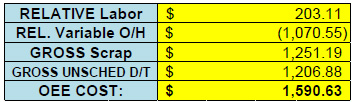Note how the ROC is negative. This is a direct result of several processes running at faster-than-target cycle times. In this table, because this is a calculation of costs, gains appear as negative values and losses as positive. If the management team prefers to change this convention then it is simply a matter of reversing the signs in the formulas. Note also that scrap and downtime are prefixed by gross, meaning they are being compared to targets of zero scrap and zero downtime. Again, this is a convention decided upon by the management team.

Over the course of a month, similar daily calculations of \$EE have been charted: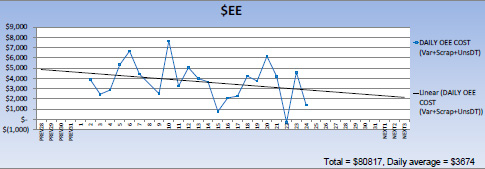At the same time, OEE was also calculated and charted: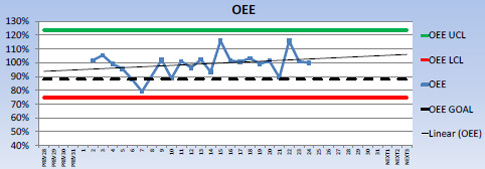Note how OEE has exceeded 100%. This is simply a result of measuring processes which have been improved beyond original business plan objectives, notably cycle times. Although unconventional, as will be discussed, the absolute value of OEE is of less concern than the actual change from one day to another.

An interesting pattern is immediately clear: as OEE increases, \$EE drops. This makes sense intuitively, and because all the data is available, it is also possible to quantify these gains and losses in terms of dollars.

Appendix 2 provides more detailed observations and analyses of the \$EE and OEE charts, including the underlying metrics from which they are calculated. In Appendix 3, \$EE and OEE data has been analyzed statistically to answer the question posed previously: What is the value of OEE? In this example, one percentage point is worth \$157. If OEE increases by 10% then operational costs improve by \$1,570 per day. These are tangible savings which derive from the budgetary overhead, scrap, and labor rates which make up \$EE.

### \$EE and the value of OEE

What is actually required for a company to reach a target OEE of 85%? Should a target even be set? How should cycle time targets be set to calculate performance efficiency? After setting up the required data collection and information systems to calculate OEE, how can employees maximize the return on investment? These are some of the questions which arise when analyzing both OEE and \$EE information.

An OEE target of 85% can be derived approximately from targets of 95% performance efficiency, 95% first-pass yield, and 95% availability, which when multiplied together result in an OEE of 85.7%. Understandably, those targets do not make sense for all businesses.

Contributing to the ambiguity is a requirement to prevent performance efficiency from exceeding 100% in order to cap OEE at 100%. What happens when processes improve and previous cycle time targets are met? The cycle target must be changed so that performance efficiency does not exceed 100%; as a consequence, OEE drops. Therefore, it is possible to manipulate OEE, but in doing so how can one process be compared objectively to another?

Instead of being too concerned about whether OEE is above or below 85%, or even whether cycle times have exceeded initial targets, by using the \$EE calculations business leaders can focus on operational metrics which have been translated into dollars and sense. By studying \$EE, the change in OEE can be interpreted readily in financial terms. The calculated value of OEE, whether greater than or less than 85%, is simply a starting point. By utilizing \$EE the leadership team can better direct resources towards reducing variation and lowering costs.

Just as each component of OEE can be charted, each factor of \$EE can be charted to understand the behavior of real costs which drive profit/loss in manufacturing. More stable inputs result in more capable outputs, reducing the daily firefighting mode and also customer complaints.

Much more can be said about data collection and analysis. Daily variation can be explained on a point-by-point basis, but over the course of time patterns emerge. With more effective measurement tools, employees can better understand variation and trends which affect their business, with the goal of tracking progress and making measureable improvements. Analyzing OEE and \$EE metrics should encourage open discussion within a company. The process, not necessarily the goal, defines a world-class leadership approach to continual improvement.

### Appendix 1: How to calculate \$EE, the financial aspect of OEE

The equation to account for overhead cost is as follows:
ROC = MACHINE RATE × RUN TIME × (ACTUAL CYCLE TIME / PLANNED CYCLE TIME – 1) (equation 1)

Note:
All factors must share the same unit of time

This equation captures the effect of cycle efficiency on overhead costs. If the actual cycle time is the same as the planned cycle time, then the entire term in brackets becomes zero, and there is neither a benefit nor a loss associated with overhead. If, on the other hand, actual cycle time is lower than planned (i.e. running faster), then the term in brackets becomes negative and the financial effect is a reduction in costs. Another way to look at this scenario of running faster is as follows: the same number of parts can be produced in less time. Therefore the benefit lies in freeing up press capacity, hence the use of machine rate as the applicable cost. On the other hand, if the actual cycle time exceeds planned cycle time then the financial effect is an increase in operating costs, hence a positive number.

Example:
Calculate the relative operating costs

Given:
MACHINE RATE = \$250/h
RUN TIME = 60min
ACTUAL CYCLE TIME = 59s
PLANNED CYCLE TIME = 60s

Solution:
ROC = \$250/h × 1h × (59/60 – 1) = –\$4.17

Therefore the relative effect of running at 59 seconds compared to the planned rate of 60 seconds is a reduction in overhead costs by \$4.17 (note the negative sign). If the same process ran at 61 seconds then the relative effect would be an increase in cost by \$4.17, the same absolute amount but a different sign, minus to plus. Savings became a loss. Another way of looking at this is one second is worth \$250/h × 1h /3600s = \$0.0694. Because there are 60 × 60 seconds in an hour, then \$0.0694 × 60 = \$4.17.

2) Relative direct labor costs (RDLC)
Similar to ROC, RDLC includes a performance efficiency term:
RDLC = LABOR RATE × RUN TIME × [(ACTUAL NO. OPERATORS – PLANNED NO. OPERATORS) + ACTUAL NO. OPERATORS × (ACTUAL CYCLE TIME / PLANNED CYCLE TIME – 1)] (equation 2)

Note:
All factors must share the same unit of time

This equation is slightly more complex than for ROC as it captures not only the effect of performance efficiency but also the effect of adding or removing labor from a work cell. If the actual number of operators is less than planned, then the first calculation in the square brackets above determines how many fewer there are and then multiplies by labor rate and the run time for a calculated savings. The second calculation in the square brackets is similar to that for ROC, and similar arguments apply. Note there can be savings or losses based on labor or cycle times, the equation will handle the different combinations.

Example:
Calculate the relative direct labor costs

Given:
LABOR RATE = \$25/h
RUN TIME = 60 min
ACTUAL NO. OPERATORS = 2
PLANNED NO. OPERATORS = 3
ACTUAL CYCLE TIME = 59s
PLANNED CYCLE TIME = 60s

Solution:
RDLC = \$25/h × 1h × [(2–3) + 2 × (59/60 – 1)] = –\$25.83

Therefore, the relative effect of reducing labor by one operator and also running at 59 seconds compared to the planned rate of 60 seconds is a reduction in relative direct labor costs by \$25.83.

3) Relative scrap cost (RSC)
Scrap cost can be calculated by the formula:
SC1 = (NO. SCRAP PARTS × PART WEIGHT × MATERIAL COST) (equation 3)

Note:
All factors must share the same unit of weight

When a scrap part is made, machine and operator times are wasted, therefore including those costs in piece price will make the calculation of financial OEE more accurate. In this case the formula will be:
SC2 = (NO. SCRAP PARTS × PIECE PRICE) (equation 4)

Example:
Calculate the scrap cost

Given:
NO. SCRAP PARTS = 2
Part weight = 10 lbs.
12
Material cost = \$1.00/lb.

Solution:
SC1 = 2 × 10 × \$1.00 = \$20.00

Therefore the scrap cost was \$20.

This simple method of calculating SC assumes the target for scrap is zero. From a business planning standpoint the plant might have internally established scrap rates that can serve as the target. In this case SC is handled differently:
RSC = (SCRAP / PRODUCTION – TARGET) × PRODUCTION × PIECE PRICE (equation 5)

Note:
RSC is the relative scrap cost

Example:
Calculate the relative scrap cost

Given:
PRODUCTION = 100 pcs
SCRAP = 10 pcs
PIECE PRICE = \$2
TARGET SCRAP = 3% (input as 0.03 in the equation)

Solution:
RSC = (10/100 – 0.03) × 100 × \$2 = \$14

Therefore the relative scrap cost was \$14. In the first example to total scrap amount was \$20, but because 3% is accounted for in the business plan, that means that 3 in 100 parts are subtracted from the 10 to leave 7, which at \$2 per piece results in a relative scrap cost of \$14.

4) Relative unscheduled downtime cost (RUDC)
Unscheduled downtime cost is calculated as follows:
UDC = UNSCHEDULED DOWNTIME × MACHINE RATE (equation 6)

Example:
Calculate the unscheduled downtime cost

Given:
UNSCHEDULED DOWNTIME = 60m
MACHINE RATE = \$250/h

Solution:
UDC = 1hr × \$250/h = \$250

Therefore the cost was \$250.

If RUDC is required, then the formula becomes slightly more complex:
RUDC = (DOWNTIME / SCHEDULED – TARGET) × SCHEDULED × RATE (equation 7)

Note:
all factors must share the same unit of time

Example:
Calculate the relative downtime

Given:
SCHEDULED time = 480m
Unscheduled DOWNTIME = 60m
Machine RATE = \$250/h
TARGET downtime = 5% (input as 0.05 in the equation)

Solution:
RUDC = (60/480 – 0.05) × 480 × \$250/60 = \$150

Therefore the relative unscheduled downtime cost was \$150. Because 5% unscheduled downtime was accounted for in the business plan, this means 480 × 0.05 × \$250, or \$100, is subtracted from the absolute downtime of \$250.

5) Other considerations
Scheduled downtime represents open capacity in manufacturing. Scheduled downtime might be used productively to perform preventive or necessary maintenance, to conduct trials, etc. which are regarded as the costs of doing business. If required, the above equations can be applied to understand the costs of those necessities and to manage them accordingly.

6) \$EE, the financial aspect of OEE
It is important to understand not only the measure of OEE, but also the financial aspects and effects on the business. As long as the management team defines how to calculate each factor in the formulas for OEE and \$EE, then the journey of improvement can begin without roadblocks.

From the financial equations (1) to (7) above:
\$EE0 = ROC + RDLC + SC + UDC (equation 8)

Or, if ALL relative costs are applied:
\$EE = ROC + RDLC + RSC + RUDC (equation 9)

Note: Both ROC and RDLC equations use planned cycle times hence the use of the term “relative,” i.e. compared to the business plan. Planned cycle time must also be greater than zero to avoid divide-by-zero errors in equations (1) and (2). Conversely, if the actual number of operators is less than planned (or zero), then equation (2) will reflect this as a cost reduction.

Key Words:
\$EE = the financial aspect of OEE
\$EE0 = the financial aspect of OEE, without all relative costs
OEE = Overall equipment effectiveness
RDLC = Relative direct labor cost
RSC = Relative scrap cost
RUDC =Relative unscheduled downtime cost
SC = Scrap cost
UDC = Unscheduled downtime cost

### Appendix 2: Detailed analysis of example

Over the course of a month, daily calculations of \$EE resulted in the following chart: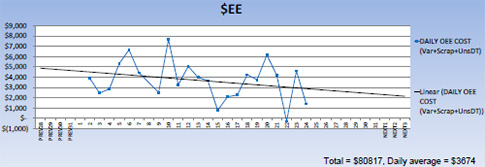At the same time, OEE was also calculated and charted:Note how OEE has been “allowed” to exceed 100%. This is simply a result of measuring processes which have been improved beyond original business plan objectives, notably cycle times. An interesting pattern is immediately clear; as OEE increases, \$EE drops. This makes sense intuitively, and because all the data is available it is also possible to quantify these gains and losses in terms of dollars

Using the charts above, OEE on Day 2 was 102%. The \$EE on this day was \$3,872. On Day 15 the OEE was 116% with a \$EE of \$769. Therefore an OEE increase of 12% corresponded with a drop in \$EE of \$3,103. On Day 7 OEE was at a low of 79%, but on this particular day \$EE was \$4,423, clearly not the highest \$EE point in the chart. Further investigation revealed higher downtime issues on a production day with fewer scheduled operating hours.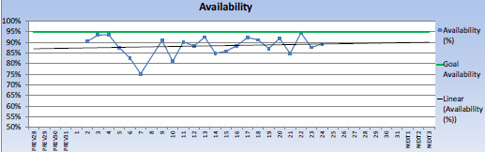On Day 10, OEE was 89% with \$EE at the highest, \$7,655. Further investigation revealed a combination of downtime and scrap issues on a heavy production day which added up to produce the highest operational costs (losses).Day 22 was a good day overall, with high OEE and \$EE below zero.

### Appendix 3: Correlation

Statistical analysis shows the following:
Pearson correlation of OEE and \$EE = –0.693, indicating a strong inverse correlation
P-Value = 0.000, indicating a significant result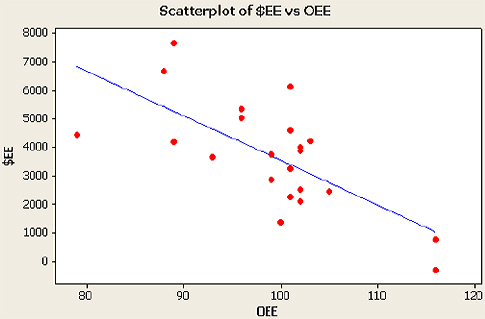Linear regression of the data produces the following equation:
\$EE = 19279 – 157 OEE (equation 3)
When OEE = 80%, \$EE = \$6,719
When OEE = 116%, \$EE = \$1,067

For this process, the slope of the line reveals 1% OEE is worth on average \$157 per day. For example, if OEE increased by 10% then operational costs would be lower by \$1,570 per day.

### Discuss### Darin Marcuz

Darin Marcuz, an engineer at Magna Exteriors molding process division, has worked in plastics manufacturing for 25 years. He is a certified Six Sigma Black Belt, holds a U.S. patent, and has a bachelor of science in chemical engineering.### Laron Colbert

Laron Colbert is an operations manager at Decostar Industries, Magna. He has worked in manufacturing for 25 years as a quality engineer, quality manager, and operations manager. Colbert is a certified Six Sigma Black Belt, and he has a bachelor of science in biology from Southern Arkansas University.

### Very interesting article

Very interesting article!

Can you please decipher the term "GROSS Unshed D/T"

### GROSS

From the article: "Note also that scrap and downtime are prefixed by gross, meaning they are being compared to targets of zero scrap and zero downtime. "

Unsched D/T = Unscheduled downtime

### I have developed OEE metrics

I have developed OEE metrics for many years and find your concept of \$EE very interesting.

However, I find your analysis of the data you present very disturbing. The use of so-called "trend lines" in your first two charts of OEE and \$EE is one of the biggest abuses of "statistical analysis" that I see all the time in meetings when someone is trying to push an agenda.  There is no trend in your data.  All you have to do is plot your data on a properly constructed Run Chart and/or a Process Behavior Chart and you will learn that you have a stable and predictable process generating \$EE and OEE numbers.  The so-called "trend lines" are just least squares fits of the data.  If you add the 95% confident interval for the line you will find that the "trend line" can have a negative slope, a positive slope, or a slope of zero.  Therefore, there IS NO TREND!!!  In addition, when I plotted your data, the r-squared of the regression is 0.069 - not significant for the degrees of freedom in your data.  You will also find that the prediction confidence interval is massively wide at all values of \$EE.

Correlation between OEE and \$EE is not necessarily causation, even if it is intuitively evident.  You show no evidence of causation.  so you end up trying to explain common cause variation is terms of special causes in a system that is clearly showing a reasonable degree of statistical control.

As W. Edwards Deming would have said about your analysis:  "Simple...obvious...and wrong."